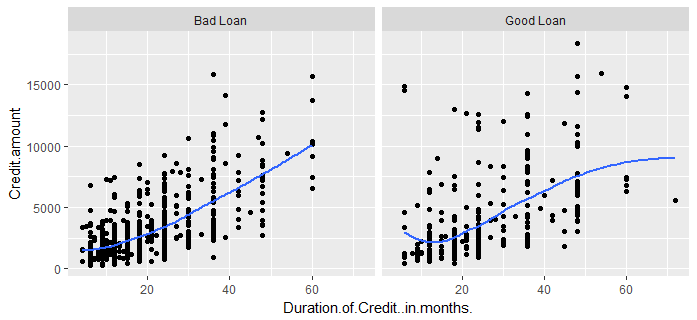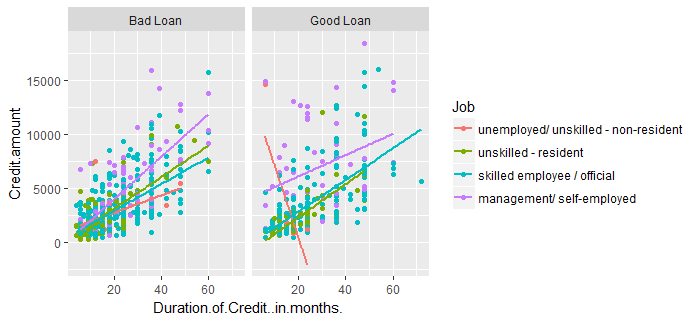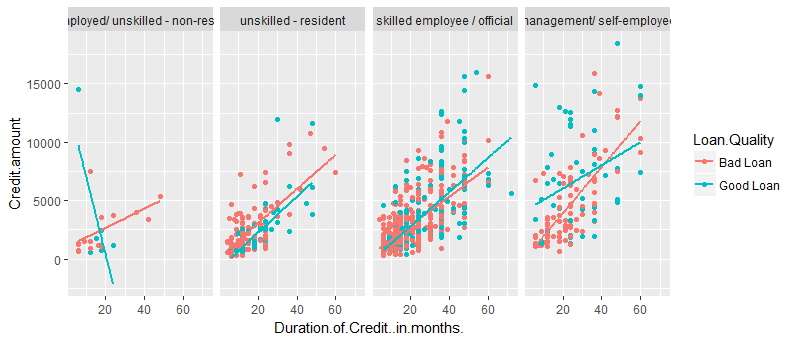# Splitting Plots with Facets in ggplots

Faceting refers to splitting the data into multiple subsets and then displaying plots for the specific subsets in a panel. Such plots are also called small-multiple plots. The facet approach partitions a plot into a matrix of panels. Each panel shows a different subset of the data.

In our scatter plot we grouped the data into bad and good loans using colors. We can use the faceting concept to split the entire graph into two separate panels one for each, good and bad loans. To do so we will use the facet_grid(. ~ ___) layer. facet_grid forms a matrix of panels defined by row and column faceting variables. facet_grid(x ~ y) will display x*y plots even if some plots are empty.

g <- ggplot(df,aes(x=Duration.of.Credit..in.months.,y=Credit.amount))
g+geom_point()+geom_smooth(se=FALSE)+
facet_grid(. ~ Loan.Quality)


We split our data only by columns. That's why we specify the rows by a simple. The columns are split by Loan.Quality. Since this variable has only two values, the graph will be split into two columns.Faceting can be specially useful when we want to bring another variable into our plot. For example in this case, we can now add the Job variable to see how the type of job of the borrower impacts the credit.

g <- ggplot(df,aes(x=Duration.of.Credit..in.months.,y=Credit.amount, color=Job))
g+geom_point()+geom_smooth(se=FALSE,method='lm')+
facet_grid(. ~ Loan.Quality)ggplot2 is beautiful and to slice the data in a different way is extremely easy. In the following graph, we exchange the two parameters Loan.Quality and Job.

g <- ggplot(df,aes(x=Duration.of.Credit..in.months.,y=Credit.amount, color=Loan.Quality))
g+geom_point()+geom_smooth(se=FALSE,method='lm')+
facet_grid(. ~ Job)